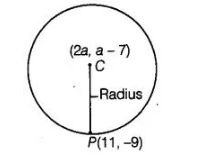# If the centre of a circle is (2a, a – 7),

Question:

If the centre of a circle is (2a, a – 7), then Find the values of a, if the  circle passes through the point (11, – 9) and has diameter 10√2 units.

Solution:

By given condition,Distance between the centre C(2a, a – 7) and the point P( 11, – 9), which lie on the circle = Radius of circle

$\therefore \quad$ Radius of circle $=\sqrt{(11-2 a)^{2}+(-9-a+7)^{2}}$ $\ldots($ i)

$\left[\because\right.$ distance between two points $\left(x_{1}, y_{1}\right)$ and $\left(x_{2}, y_{2}\right)=\sqrt{\left.\left(x_{2}-x_{1}\right)^{2}+\left(y_{2}-y_{1}\right)^{2}\right]}$

Given that, $\quad$ length of diameter $=10 \sqrt{2}$

$\therefore$ Length of radius $=\frac{\text { Length of diameter }}{2}$

$=\frac{10 \sqrt{2}}{2}=5 \sqrt{2}$

Put this value in Eq. (i), we get $5 \sqrt{2}=\sqrt{(11-2 a)^{2}+(-2-a)^{2}}$

Squaring on both sides, we get

$50=(11-2 a)^{2}+(2+a)^{2}$

$\Rightarrow \quad 50=121+4 a^{2}-44 a+4+a^{2}+4 a$

$\Rightarrow \quad 5 a^{2}-40 a+75=0$

$\Rightarrow \quad a^{2}-8 a+15=0$

$\Rightarrow \quad a^{2}-5 a-3 a+15=0$ [by factorisation method]

$\Rightarrow \quad a(a-5)-3(a-5)=0$

$\therefore \quad a=3,5$

Hence, the required values of a are 5 and 3.